#### Geometry

###### back to index

Quadrilateral $APBQ$ is inscribed in circle $\omega$ with $angle P = \angle Q = 90^{\circ}$ and $AP = AQ < BP$. Let $X$ be a variable point on segment $\overline{PQ}$. Line $AX$ meets $\omega$ again at $S$ (other than $A$). Point $T$ lies on arc $AQB$ of $\omega$ such that $\overline{XT}$ is perpendicular to $\overline{AX}$. Let $M$ denote the midpoint of chord $\overline{ST}$. As $X$ varies on segment $\overline{PQ}$, show that $M$ moves along a circle.

Point $B$ lies on line segment $\overline{AC}$ with $AB=16$ and $BC=4$. Points $D$ and $E$ lie on the same side of line $AC$ forming equilateral triangles $\triangle ABD$ and $\triangle BCE$. Let $M$ be the midpoint of $\overline{AE}$, and $N$ be the midpoint of $\overline{CD}$. The area of $\triangle BMN$ is $x$. Find $x^2$.

Point $A,B,C,D,$ and $E$ are equally spaced on a minor arc of a cirle. Points $E,F,G,H,I$ and $A$ are equally spaced on a minor arc of a second circle with center $C$ as shown in the figure below. The angle $\angle ABD$ exceeds $\angle AHG$ by $12^\circ$. Find the degree measure of $\angle BAG$.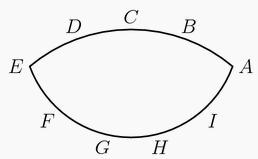In the diagram below, $ABCD$ is a square. Point $E$ is the midpoint of $\overline{AD}$. Points $F$ and $G$ lie on $\overline{CE}$, and $H$ and $J$ lie on $\overline{AB}$ and $\overline{BC}$, respectively, so that $FGHJ$ is a square. Points $K$ and $L$ lie on $\overline{GH}$, and $M$ and $N$ lie on $\overline{AD}$ and $\overline{AB}$, respectively, so that $KLMN$ is a square. The area of $KLMN$ is $99$. Find the area of $FGHJ$.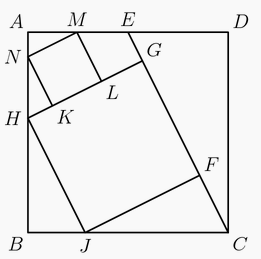Triangle $ABC$ has positive integer side lengths with $AB=AC$. Let $I$ be the intersection of the bisectors of $\angle B$ and $\angle C$. Suppose $BI=8$. Find the smallest possible perimeter of $\triangle ABC$.

A block of wood has the shape of a right circular cylinder with radius $6$ and height $8$, and its entire surface has been painted blue. Points $A$ and $B$ are chosen on the edge of one of the circular faces of the cylinder so that $\overset\frown{AB}$ on that face measures $120^\text{o}$. The block is then sliced in half along the plane that passes through point $A$, point $B$, and the center of the cylinder, revealing a flat, unpainted face on each half. The area of one of these unpainted faces is $a\cdot\pi + b\sqrt{c}$, where $a$, $b$, and $c$ are integers and $c$ is not divisible by the square of any prime. Find $a+b+c$.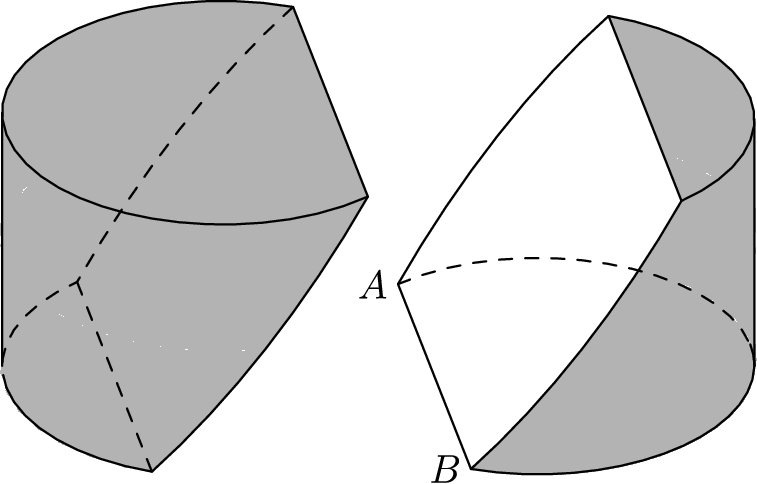Triangle $ABC$ has side lengths $AB = 12$, $BC = 25$, and $CA = 17$. Rectangle $PQRS$ has vertex $P$ on $\overline{AB}$, vertex $Q$ on $\overline{AC}$, and vertices $R$ and $S$ on $\overline{BC}$. In terms of the side length $PQ = w$, the area of $PQRS$ can be expressed as the quadratic polynomial Area($PQRS$) = $\alpha w - \beta \cdot w^2$. Then the coefficient $\beta = \frac{m}{n}$, where $m$ and $n$ are relatively prime positive integers. Find $m+n$.

A cylindrical barrel with radius $4$ feet and height $10$ feet is full of water. A solid cube with side length $8$ feet is set into the barrel so that the diagonal of the cube is vertical. The volume of water thus displaced is $v$ cubic feet. Find $v^2$.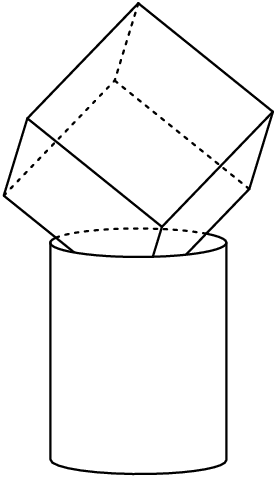The circumcircle of acute $\triangle ABC$ has center $O$. The line passing through point $O$ perpendicular to $\overline{OB}$ intersects lines $AB$ and $BC$ and $P$ and $Q$, respectively. Also $AB=5$, $BC=4$, $BQ=4.5$, and $BP=\frac{m}{n}$, where $m$ and $n$ are relatively prime positive integers. Find $m+n$.

Circles $\mathcal{P}$ and $\mathcal{Q}$ have radii $1$ and $4$, respectively, and are externally tangent at point $A$. Point $B$ is on $mathcal{P}$ and point $C$ is on $\mathcal{Q}$ so that line $BC$ is a common external tangent of the two circles. A line $\ell$ through $A$ intersects $\mathcal{P}$ again at $D$ and intersects $\mathcal{Q}$ again at $E$. Points $B$ and $C$ lie on the same side of $\ell$, and the areas of $\triangle DBA$ and $\triangle ACE$ are equal. This common area is $\frac{m}{n}$, where $m$ and $n$ are relatively prime positive integers. Find $m+n$.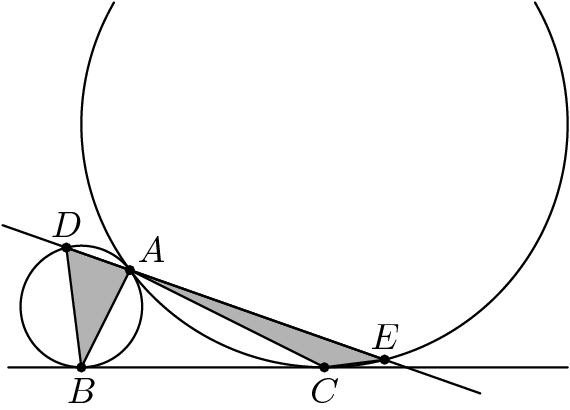The graphs $y=3(x-h)^2+j$ and $y=2(x-h)^2+k$ have y-intercepts of $2013$ and $2014$, respectively, and each graph has two positive integer x-intercepts. Find $h$.

A disk with radius $1$ is externally tangent to a disk with radius $5$. Let $A$ be the point where the disks are tangent, $C$ be the center of the smaller disk, and $E$ be the center of the larger disk. While the larger disk remains fixed, the smaller disk is allowed to roll along the outside of the larger disk until the smaller disk has turned through an angle of $360^\circ$. That is, if the center of the smaller disk has moved to the point $D$, and the point on the smaller disk that began at $A$ has now moved to point $B$, then $\overline{AC}$ is parallel to $\overline{BD}$. Then $sin^2(\angle BEA)=\tfrac{m}{n}$, where $m$ and $n$ are relatively prime positive integers. Find $m+n$.

On square $ABCD$, points $E,F,G$, and $H$ lie on sides $\overline{AB},\overline{BC},\overline{CD},$ and $\overline{DA},$ respectively, so that $\overline{EG} \perp \overline{FH}$ and $EG=FH = 34$. Segments $\overline{EG}$ and $\overline{FH}$ intersect at a point $P$, and the areas of the quadrilaterals $AEPH, BFPE, CGPF,$ and $DHPG$ are in the ratio $269:275:405:411.$ Find the area of square $ABCD$.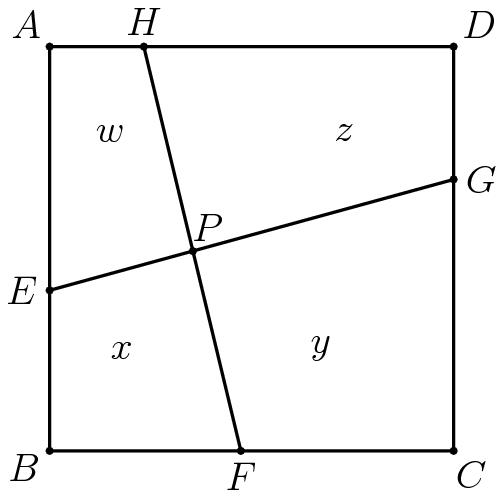In $\triangle ABC$, $AB = 3$, $BC = 4$, and $CA = 5$. Circle $\omega$ intersects $\overline{AB}$ at $E$ and $B$, $\overline{BC}$ at $B$ and $D$, and $overline{AC}$ at $F$ and $G$. Given that $EF=DF$ and $\frac{DG}{EG} = \frac{3}{4}$, length $DE=\frac{a\sqrt{b}}{c}$, where $a$ and $c$ are relatively prime positive integers, and $b$ is a positive integer not divisible by the square of any prime. Find $a+b+c$.

A rectangle has sides of length $a$ and 36. A hinge is installed at each vertex of the rectangle, and at the midpoint of each side of length 36. The sides of length $a$ can be pressed toward each other keeping those two sides parallel so the rectangle becomes a convex hexagon as shown. When the figure is a hexagon with the sides of length $a$ parallel and separated by a distance of 24, the hexagon has the same area as the original rectangle. Find $a^2$.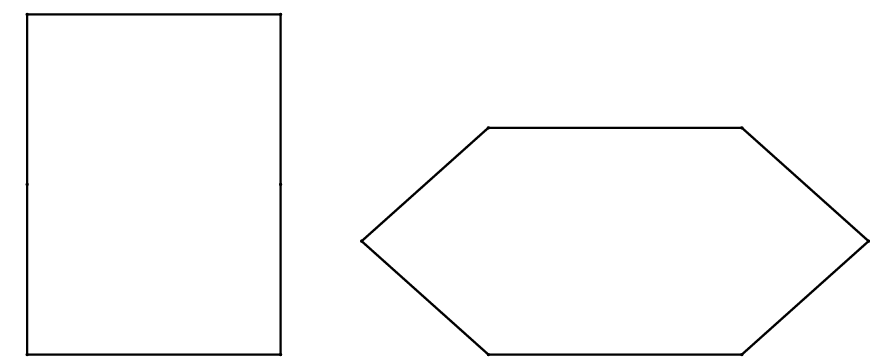Circle $C$ with radius 2 has diameter $\overline{AB}$. Circle D is internally tangent to circle $C$ at $A$. Circle $E$ is internally tangent to circle $C$, externally tangent to circle $D$, and tangent to $\overline{AB}$. The radius of circle $D$ is three times the radius of circle $E$, and can be written in the form $\sqrt{m}-n$, where $m$ and $n$ are positive integers. Find $m+n$.

In $\triangle RED$, $\angle DRE=75^{\circ}$ and $\angle RED=45^{\circ}$. $|RD|=1$. Let $M$ be the midpoint of segment $\overline{RD}$. Point $C$ lies on side $\overline{ED}$ such that $\overline{RC}\perp\overline{EM}$. Extend segment $\overline{DE}$ through $E$ to point $A$ such that $CA=AR$. Then $AE=\frac{a-\sqrt{b}}{c}$, where $a$ and $c$ are relatively prime positive integers, and $b$ is a positive integer. Find $a+b+c$.

In $\triangle{ABC}, AB=10, \angle{A}=30^{\circ}$, and $\angle{C=45^{\circ}}$. Let $H, D,$ and $M$ be points on the line $BC$ such that $AH\perp{BC}$, $\angle{BAD}=\angle{CAD}$, and $BM=CM$. Point $N$ is the midpoint of the segment $HM$, and point $P$ is on ray $AD$ such that $PN\perp{BC}$. Then $AP^2=\dfrac{m}{n}$, where $m$ and $n$ are relatively prime positive integers. Find $m+n$.

Let $ABCD$ be a square, and let $E$ and $F$ be points on $\overline{AB}$ and $\overline{BC},$ respectively. The line through $E$ parallel to $\overline{BC}$ and the line through $F$ parallel to $\overline{AB}$ divide $ABCD$ into two squares and two nonsquare rectangles. The sum of the areas of the two squares is $\frac{9}{10}$ of the area of square $ABCD.$ Find $\frac{AE}{EB} + \frac{EB}{AE}.$

A rectangular box has width $12$ inches, length $16$ inches, and height $\frac{m}{n}$ inches, where $m$ and $n$ are relatively prime positive integers. Three faces of the box meet at a corner of the box. The center points of those three faces are the vertices of a triangle with an area of $30$ square inches. Find $m+n$.

A paper equilateral triangle $ABC$ has side length $12$. The paper triangle is folded so that vertex $A$ touches a point on side $\overline{BC}$ a distance $9$ from point $B$. The length of the line segment along which the triangle is folded can be written as $\frac{m\sqrt{p}}{n}$, where $m$, $n$, and $p$ are positive integers, $m$ and $n$ are relatively prime, and $p$ is not divisible by the square of any prime. Find $m+n+p$.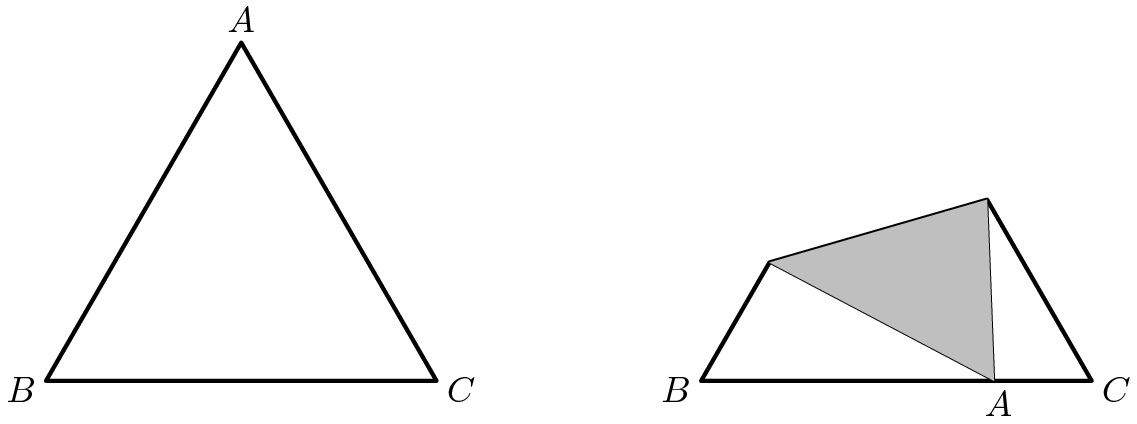Let $\bigtriangleup PQR$ be a triangle with $\angle P = 75^\circ$ and $\angle Q = 60^\circ$. A regular hexagon $ABCDEF$ with side length 1 is drawn inside $\triangle PQR$ so that side $\overline{AB}$ lies on $\overline{PQ}$, side $\overline{CD}$ lies on $\overline{QR}$, and one of the remaining vertices lies on $\overline{RP}$. There are positive integers $a, b, c,$ and $d$ such that the area of $\triangle PQR$ can be expressed in the form $\frac{a+b\sqrt{c}}{d}$, where $a$ and $d$ are relatively prime, and c is not divisible by the square of any prime. Find $a+b+c+d$.

Triangle $AB_0C_0$ has side lengths $AB_0 = 12$, $B_0C_0 = 17$, and $C_0A = 25$. For each positive integer $n$, points $B_n$ and $C_n$ are located on $\overline{AB_{n-1}}$ and $\overline{AC_{n-1}}$, respectively, creating three similar triangles $\triangle AB_nC_n \sim \triangle B_{n-1}C_nC_{n-1} \sim \triangle AB_{n-1}C_{n-1}$. The area of the union of all triangles $B_{n-1}C_nB_n$ for $n\geq1$ can be expressed as $\tfrac pq$, where $p$ and $q$ are relatively prime positive integers. Find $q$.

In the Cartesian plane let $A = (1,0)$ and $B = \left( 2, 2\sqrt{3} \right)$. Equilateral triangle $ABC$ is constructed so that $C$ lies in the first quadrant. Let $P=(x,y)$ be the center of $\triangle ABC$. Then $x \cdot y$ can be written as $\tfrac{p\sqrt{q}}{r}$, where $p$ and $r$ are relatively prime positive integers and $q$ is an integer that is not divisible by the square of any prime. Find $p+q+r$.

In equilateral $\triangle ABC$ let points $D$ and $E$ trisect $\overline{BC}$. Then $\sin(\angle DAE)$ can be expressed in the form $\frac{a\sqrt{b}}{c}$, where $a$ and $c$ are relatively prime positive integers, and $b$ is an integer that is not divisible by the square of any prime. Find $a+b+c$.